DraftKings 2019 Big 12 Odds
+100
1 to 1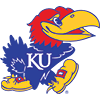Kansas
42.5% implied probability

+300
3 to 1Baylor
21.3% implied probability

+400
4 to 1West Virginia
17.0% implied probability

+800
8 to 1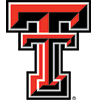Texas Tech
9.4% implied probability

+1800
18 to 1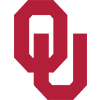Oklahoma
4.5% implied probability

+4000
40 to 1Texas
2.1% implied probability

+5000
50 to 1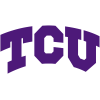TCU
1.7% implied probability

+15000
150 to 1Kansas State
0.6% implied probability

+15000
150 to 1Oklahoma State
0.6% implied probability

+20000
200 to 1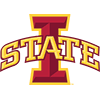Iowa State
0.4% implied probability